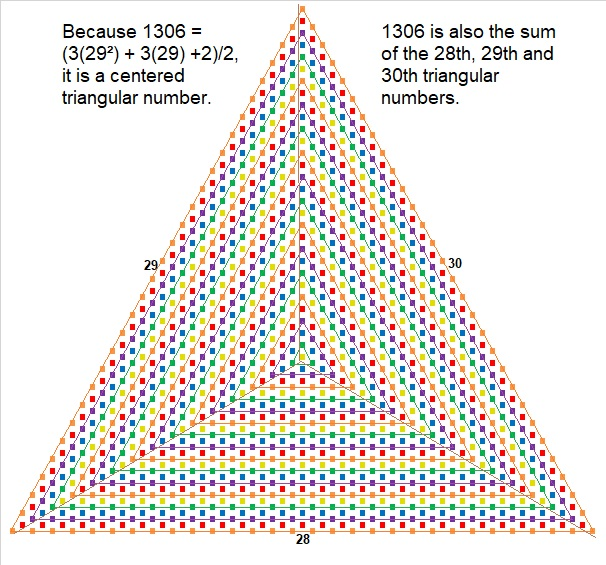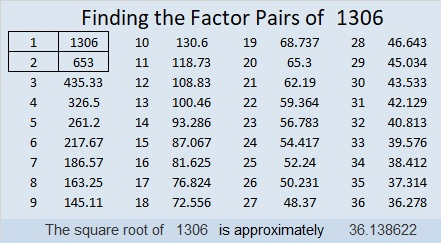# 1306 is a Centered Triangular Number

1306 tiny squares can be arranged in a triangle as I have done in the graphic below. This arrangement makes 1306 a centered triangular number:• 1306 is a composite number.
• Prime factorization: 1306 = 2 × 653
• The exponents in the prime factorization are 1 and 1. Adding one to each and multiplying we get (1 + 1)(1 + 1) = 2 × 2 = 4. Therefore 1306 has exactly 4 factors.
• Factors of 1306: 1, 2, 653, 1306
• Factor pairs: 1306 = 1 × 1306 or 2 × 653
• 1306 has no square factors that allow its square root to be simplified. √1306 ≈ 36.13862OEIS.org informs us that 1306 = 1¹ + 3² + 0³ + 6⁴.

1306 is the sum of two squares:
35² + 9² = 1306

1306 is the hypotenuse of a Pythagorean triple:
630-1144-1306 calculated from 2(35)(9), 35² – 9², 35² + 9²

This site uses Akismet to reduce spam. Learn how your comment data is processed.